# Solving Linear Inequalities and Compound Inequalities Inequality Symbols

• Slides: 12Solving Linear Inequalities and Compound Inequalities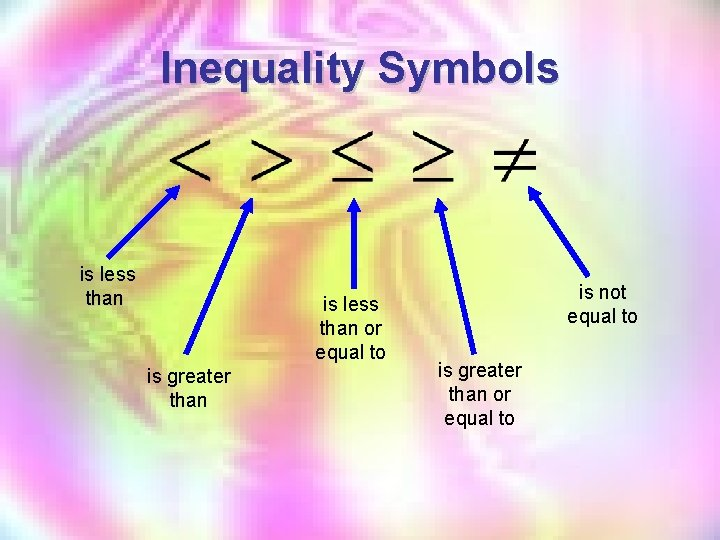Inequality Symbols is less than or equal to is greater than is not equal to is greater than or equal to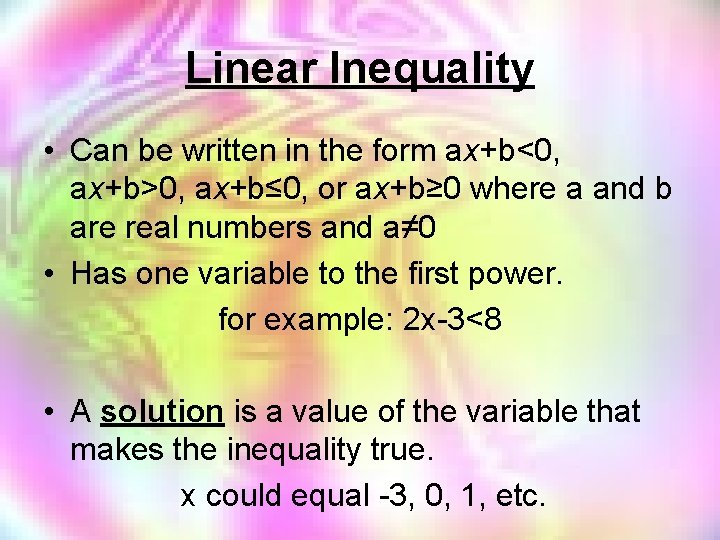Linear Inequality • Can be written in the form ax+b<0, ax+b>0, ax+b≤ 0, or ax+b≥ 0 where a and b are real numbers and a≠ 0 • Has one variable to the first power. for example: 2 x-3<8 • A solution is a value of the variable that makes the inequality true. x could equal -3, 0, 1, etc.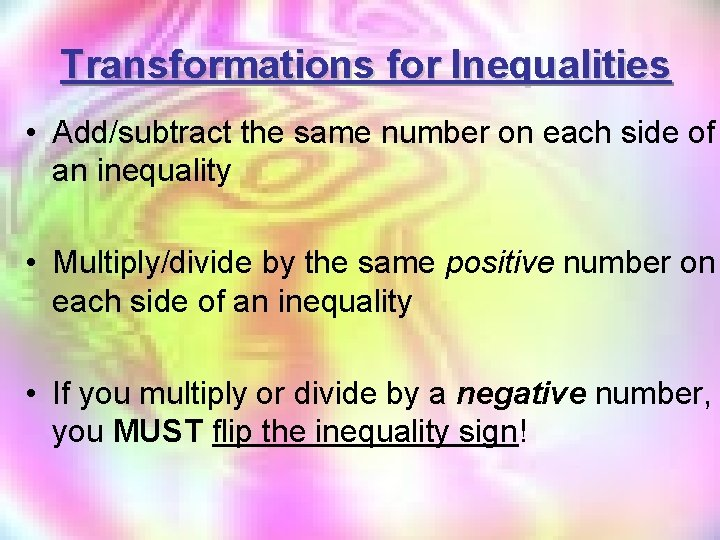Transformations for Inequalities • Add/subtract the same number on each side of an inequality • Multiply/divide by the same positive number on each side of an inequality • If you multiply or divide by a negative number, you MUST flip the inequality sign!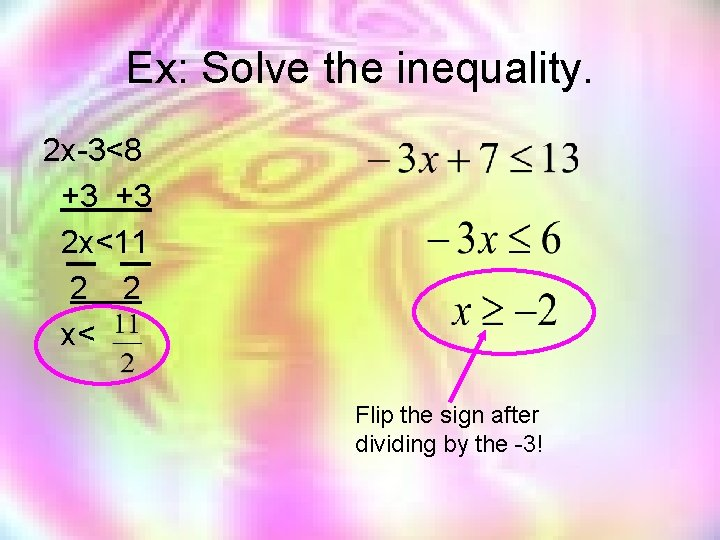Ex: Solve the inequality. 2 x-3<8 +3 +3 2 x<11 2 2 x< Flip the sign after dividing by the -3!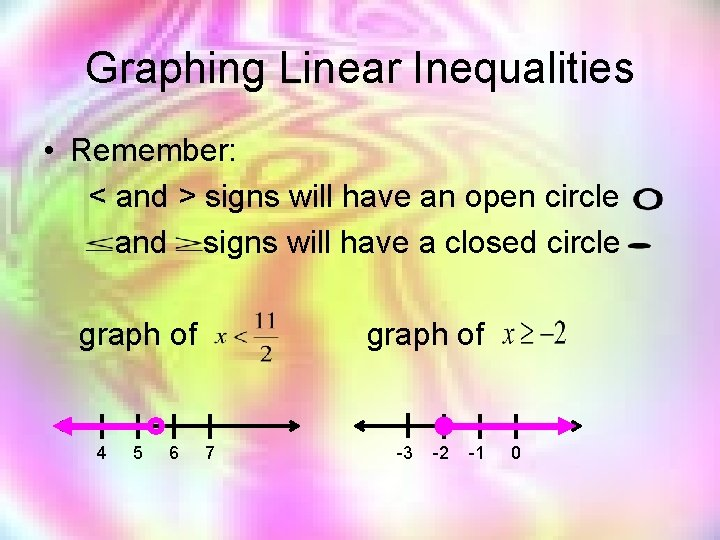Graphing Linear Inequalities • Remember: < and > signs will have an open circle and signs will have a closed circle graph of 4 5 6 graph of 7 -3 -2 -1 0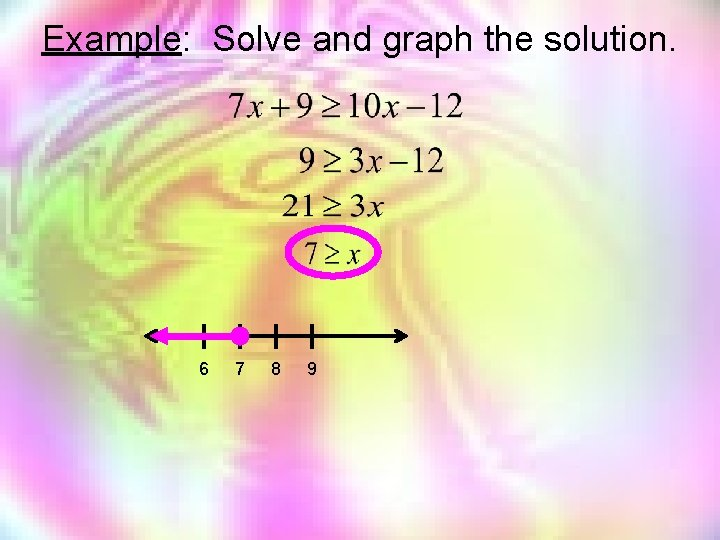Example: Solve and graph the solution. 6 7 8 9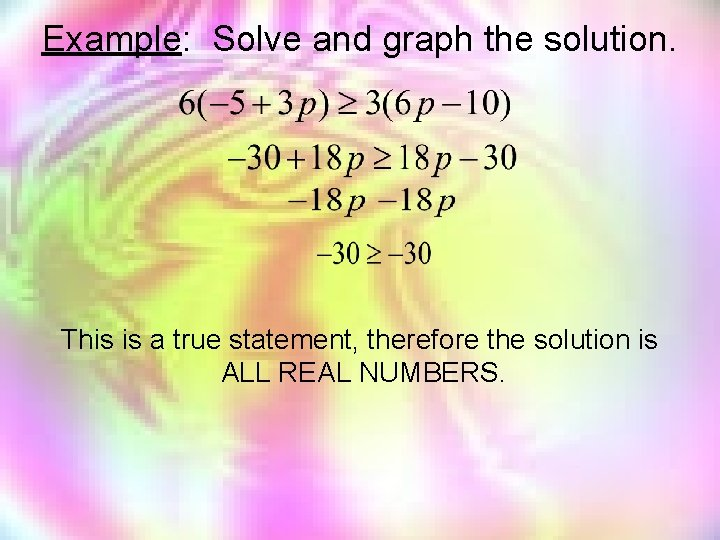Example: Solve and graph the solution. This is a true statement, therefore the solution is ALL REAL NUMBERS.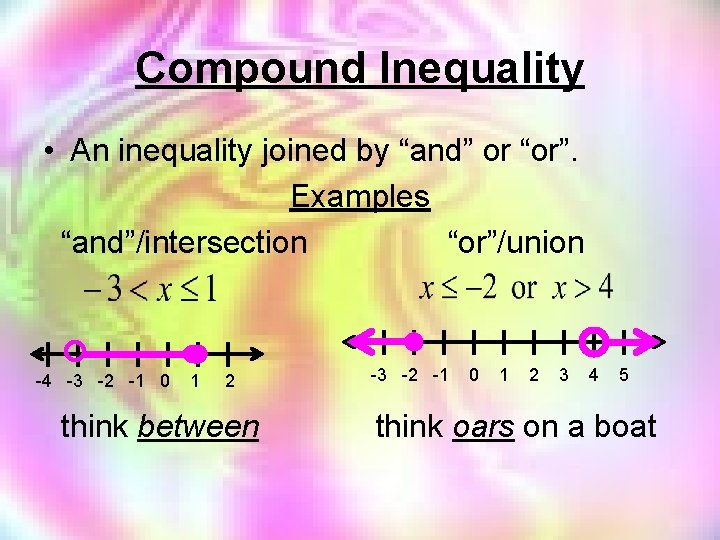Compound Inequality • An inequality joined by “and” or “or”. Examples “and”/intersection “or”/union -4 -3 -2 -1 0 1 2 think between -3 -2 -1 0 1 2 3 4 5 think oars on a boatExample: Solve & graph. -9 < t+4 < 10 -4 -4 -4 -13 < t < 6 Think between! -13 6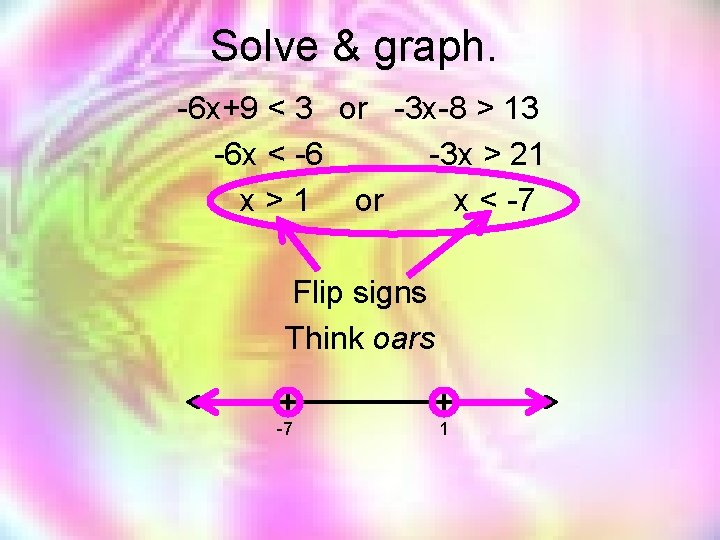Solve & graph. -6 x+9 < 3 or -3 x-8 > 13 -6 x < -6 -3 x > 21 x > 1 or x < -7 Flip signs Think oars -7 1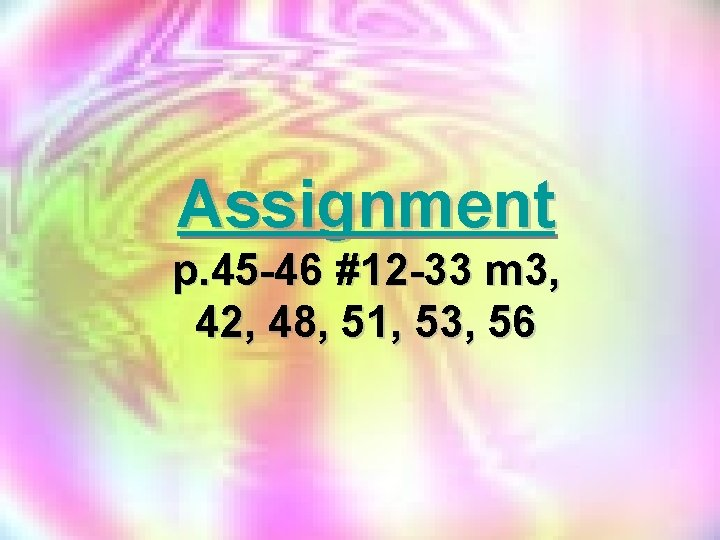Assignment p. 45 -46 #12 -33 m 3, 42, 48, 51, 53, 56